IMO Shortlist 2010 problem G3

Kvaliteta:
Avg: 3,0
Težina:
Avg: 7,0
Let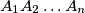$A_1A_2 \ldots A_n$ be a convex polygon. Point$P$ inside this polygon is chosen so that its projections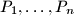$P_1, \ldots , P_n$ onto lines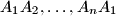$A_1A_2, \ldots , A_nA_1$ respectively lie on the sides of the polygon. Prove that for arbitrary points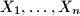$X_1, \ldots , X_n$ on sides$A_1A_2, \ldots , A_nA_1$ respectively,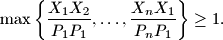Proposed by Nairi Sedrakyan, Armenia
Izvor: Međunarodna matematička olimpijada, shortlist 2010